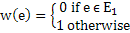# GATE | GATE-CS-2003 | Question 67

Let G = (V, E) be an undirected graph with a subgraph G1 = (V1, El). Weights are assigned to edges of G as follows :

``````

A single-source shortest path algorithm is executed on the weighted graph (V, E, w) with an arbitrary vertex ν1 of V1 as the source. Which of the following can always be inferred from the path costs computed?
(A) The number of edges in the shortest paths from ν1 to all vertices of G
(B) G1 is connected
(C) V1 forms a clique in G
(D) G1 is a tree